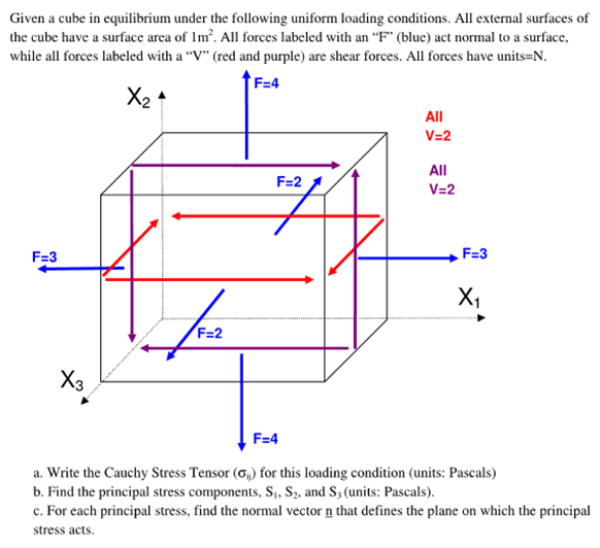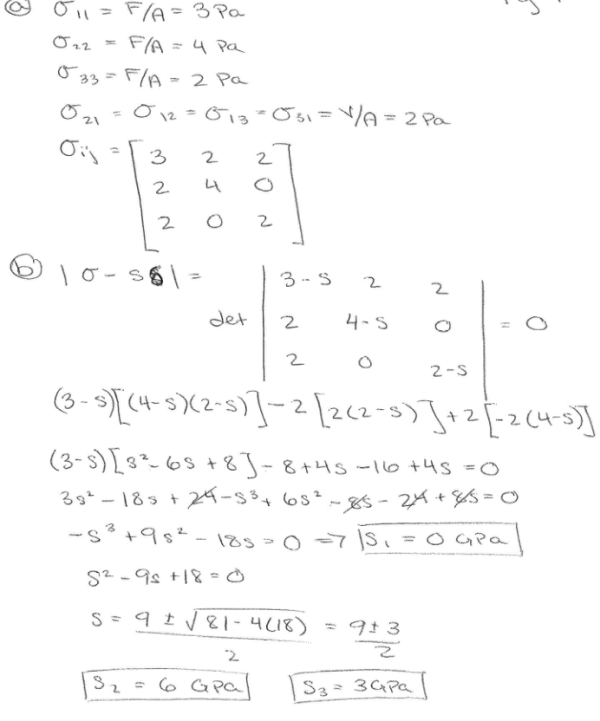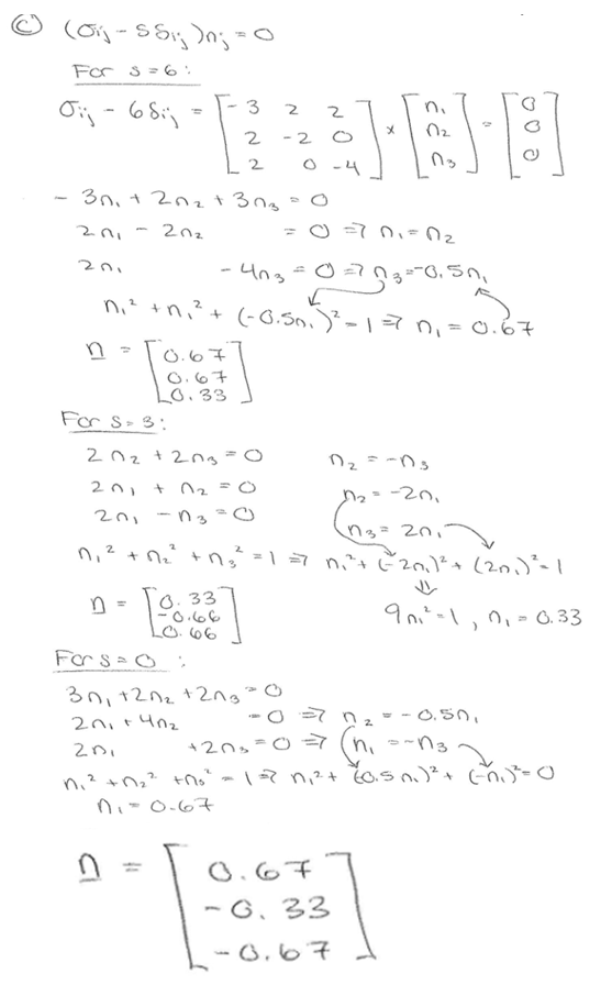Given a cube in equilibrium under the following uniform loading conditions. All external surfaces of teh cube have a surface area of 1 m^2. All forces labeled with an F (blue) act normal to a surface, while all forces labeled with a V (red and purple) are shear forces. All forces have units=N. a) Write the Cauchy Stress Tensor for this loading condition. b) Find the prinicpal stress components S1, S2, and S3. c) For each principal stress, find the normal vector n that defines the plane on which the principal stress acts.Given a cube in equilibrium under the following uniform loading conditions. All external surfaces of teh cube have a surface area of 1 m^2. All forces labeled with an F (blue) act normal to a surface, while all forces labeled with a V (red and purple) are shear forces. All forces have units=N. a) Write the Cauchy Stress Tensor for this loading condition. b) Find the prinicpal stress components S1, S2, and S3. c) For each principal stress, find the normal vector n that defines the plane on which the principal stress acts.

biomechanics page 1 biomechanics biomechanics biomechanics biomechanics biomechanics biomechanics biomechanics biomechanics page 2